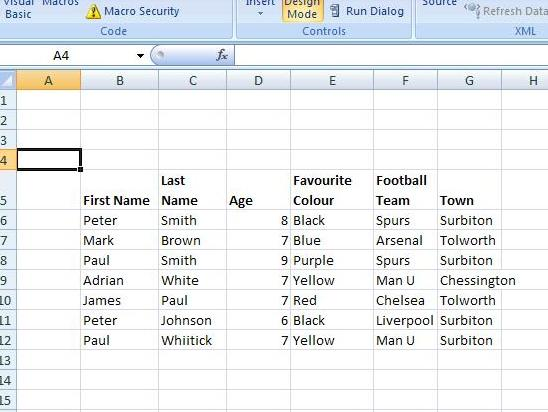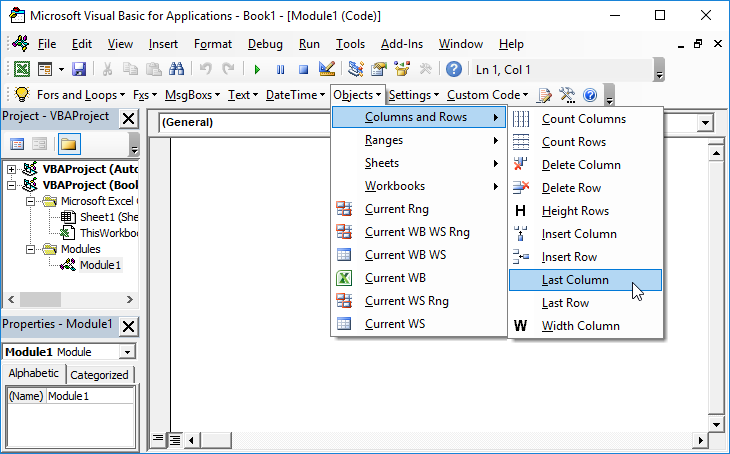# Vlookup Multiple Criteria with VBA UDF

## Vlookup Multiple Conditions Using VBA

Consider the following data table:The standard Vlookup function within Excel has the following format:

VLOOKUP(“”Mark”, B6:G12”,2,FALSE)

Which will return “Brown”.

However, what about if we wanted to look up on 2 or more conditions e.g the first name, last name and the age in the above table ? The following UDF allows us to do this:

``````Function ThreeParameterVlookup(Data_Range As Range, Col As Integer, Parameter1 As Variant, Parameter2 As Variant, Parameter3 As Variant) As Variant
'Declare Variables
Dim Cell
Dim Current_Row As Integer
Dim No_Of_Rows_in_Range As Integer
Dim No_of_Cols_in_Range As Integer
Dim Matching_Row As Integer

'set answer to N/A by default
ThreeParameterVlookup = CVErr(xlErrNA)
Matching_Row = 0
Current_Row = 1

No_Of_Rows_in_Range = Data_Range.Rows.Count
No_of_Cols_in_Range = Data_Range.Columns.Count

'Check if Col is greater than number of columns in range

If (Col > No_of_Cols_in_Range) Then
ThreeParameterVlookup = CVErr(xlErrRef)
End If
If (Col <= No_of_Cols_in_Range) Then
Do
If ((Data_Range.Cells(Current_Row, 1).Value = Parameter1) And _
(Data_Range.Cells(Current_Row, 2).Value = Parameter2) And _
(Data_Range.Cells(Current_Row, 3).Value = Parameter3)) Then
Matching_Row = Current_Row

End If
Current_Row = Current_Row + 1
Loop Until ((Current_Row = No_Of_Rows_in_Range) Or (Matching_Row <> 0))

If Matching_Row <> 0 Then
ThreeParameterVlookup = Data_Range.Cells(Matching_Row, Col)
End If

End If

End Function``````

It has the following syntax:

ThreeParameterVlookup(Data_Range, Col , Parameter1, Parameter2 , Parameter3 )

Where:
• Data_Range is the range of the data
• Col is an integer for the required column
• Parameter1, Parameter2 and Parameter3 are the values from the first three columns respectively

So that:

=ThreeParameterVlookup(B6:G12,6,”Mark”,”Brown”,7) will return ”Tolworth” as this is a match on “Mark”, “Brown”, and 7 and a reference to the 6th column

Note that this function will also worked with (dynamic) named ranges as well:

=ThreeParameterVlookup(named_range,6,”Adrian”,”White”,7) will return “Chessington” where we have set up the named range “Named_Range”.

If Excel can’t locate a match then “N/A” is returned by default. In fact, the function assumes a value of N/A at the outset and then only changes when it finds an exact match.

Also if the value of Col exceeds the number of columns then a Reference error occurs.